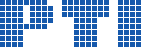Polish Information Processing SocietyAccuracy Evaluation of Classical Integer Order Based and Direct Non-integer Order Numerical Algorithms of Non-integer Order Derivatives and Integrals Computations

Dariusz W. Brzeziński, Piotr Ostalczyk

Citation: Proceedings of the 2014 Federated Conference on Computer Science and Information Systems, M. Ganzha, L. Maciaszek, M. Paprzycki (eds). ACSIS, Vol. 2, pages 553560 ()

Full text

Abstract. In this paper the authors evaluate in context of numerical calculations accuracy classical integer order and direct non-integer based order numerical algorithms of non-integer orders derivatives and integrals computations. Classical integer order based algorithm involves integer and fractional order differentiation and integration operators concatenation to obtain non-integer order. Riemann-Liouville and Caputo formulas are applied to obtain directly derivatives and integrals of non-integer orders. The following accuracy comparison analysis enables to answer the question, which algorithm of the two is burdened with lower computational error. The accuracy is estimated applying non-integer order derivatives and integrals computational formulas of some elementary functions available in the literature of the subject.